3D Pie Plot Helper VI

LabVIEW 2018 Help

Edition Date: March 2018
Part Number: 371361R-01
View Product InfoLabVIEW 2016 HelpLabVIEW 2017 HelpLabVIEW 2018 HelpLabVIEW 2019 HelpLabVIEW 2020 Help

Requires: Full Development System

Generates a pie chart.Note  This VI appears on the block diagram when you add a Pie plot to the front panel. You can find the Pie plot on the 3D Graph palette.

Example

Use the pull-down menu to select an instance of this VI.

 Select an instance VectorMatrix

Vector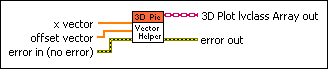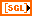x vector is a 1D array that specifies the size of the slices, or percentages of pie, that appear in the graph. LabVIEW ignores the non-positive elements in x vector.offset vector specifies how far slices of pie offset from the center point if they offset. The length of offset vector must equal the length of x vector.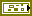error in describes error conditions that occur before this node runs. This input provides standard error in functionality.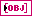3D Plot lvclass Array out is the graph of the 3D plot.error out contains error information. This output provides standard error out functionality.

Matrix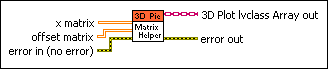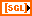x matrix is a 2D array that specifies the size of the slices, or percentages of pie, that appear in the graph. LabVIEW ignores the non-positive elements in x matrix.offset matrix specifies how far slices of pie offset from the center point if they offset. The length of offset matrix must equal the length of x matrix.error in describes error conditions that occur before this node runs. This input provides standard error in functionality.3D Plot lvclass Array out is the graph of the 3D plot.error out contains error information. This output provides standard error out functionality.

Example

Refer to the 3D Pie Graph VI in the labview\examples\Controls and Indicators\Graphs and Charts\Math Plots - 3D directory for an example of using the 3D Pie Plot Helper VI.